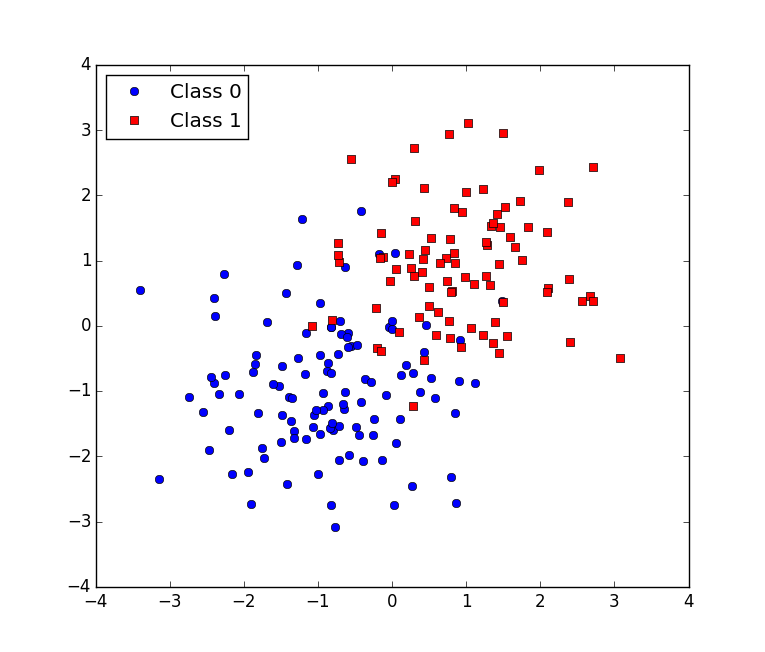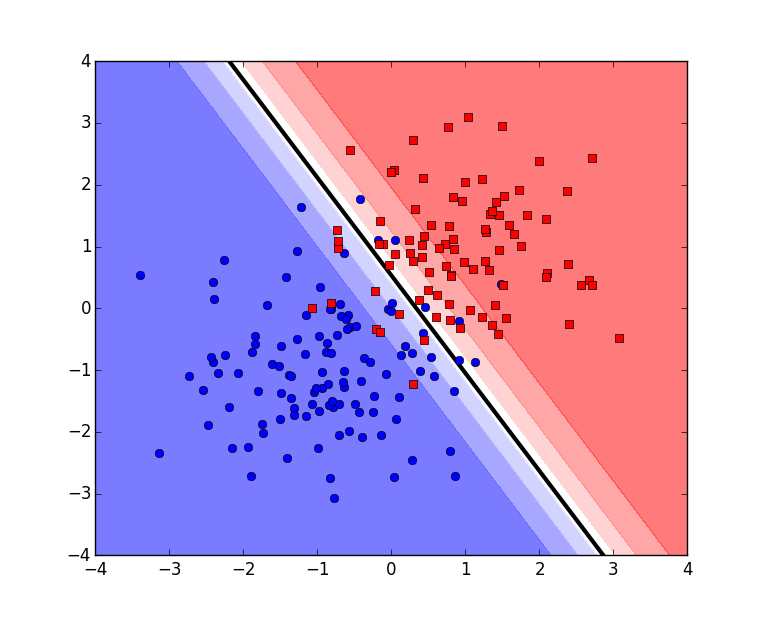This post is a continuation of the previous introduction to binary classification. I’ll go over some Python code to implement the logistic model discussed in the previous post. We’ll use simple gradient ascent to find the model parameters.

### Sample data

Consider $p=2$, $P(Y=1)=0.6$, and $P(X|Y=j) \sim \mathcal{N}(\mu_j, I)$ where $I$ is the identity matrix and $\mu_0 = \begin{bmatrix} -1 & -1 \end{bmatrix}^T$, $\mu_1 = \begin{bmatrix} 1 & 1 \end{bmatrix}^T$. We can generate some sample data with $n=200$ (realizations of the random variables $\mathbf{X}$ and $\mathbf{Y}$ mentioned in the previous post) using the following:

…and we can plot the data with:

which generates:Visually, we can see the data should be able to be separated by a line fairly well.

### Partial derivatives

We assume the parameters are independent. We assume $b$ is uniform with a large range (no regularization), and $w$ are gaussian with variance $1/\lambda$. So,

\begin{align*} D_bl(w,b) &= \sum_{i=1}^n y^i - g(x^i|w,b) =: \sum_{i=1}^n (Y-G)_i \\ D_{w_k}l(w,b) &= -\lambda w_k + \sum_{i=1} (y^i - g(x^i|w,b))x_k^i \\ D_wl(w,b) &= -\lambda w + X^T(Y-G) \end{align*}

The definitions of the vectors, $G$ and $Y$, allow us to compute the gradient with respect to all of $w$ more efficiently using the matrix-vector product. Note that $\lambda$ is a free regularization parameter to be set. Finally, we take gradient ascent steps from some initial parameters using a fixed step size, $\eta$:

\begin{align*} b &\leftarrow b + \eta \cdot D_bl(w,b) \\ w &\leftarrow w + \eta \cdot D_wl(w,b) \end{align*}

Let’s implement this:

and write a little test suite:

This outputs:

It is very important to test the model on a data set held out from the parameter learning process. Although it is fine to monitor the progress of the learning process using the data set used for learning parameters, scores for the model should not be reported using this data unless it is made completely explicit. It is the scores on data not used to learn parameters that provide insight as to how the model might generalize to unseen data. Thus, these scores are more truthful to report.

Note that I ignored the regularization term. It turns out to be not significant for this toy problem (try it yourself). However, in problems where $n$ is small, $p$ is large, or both, regularization can help tremendously.

Finally let’s visualize the predictions made over the region and the decision boundary:

This produces:And that’s all. You can download all the above scripts in one file here.